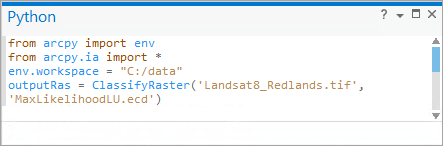# 快速浏览：在 Image Analyst 中使用地图代数

``````from arcpy.ia import *
elevationPlus100 = Plus("inelevation", 100)``````

## 运行“地图代数”的基础知识

• 栅格计算器工具
• Python 窗口
• 偏好的 Python 集成开发环境 (IDE)

### Python 窗口

Python 窗口中，可以高效、便捷地使用地理处理工具和 ArcGIS 中的 Python 函数。可在该窗口中运行的 Python 命令包括单行代码，也包括带逻辑的复杂块。在 Python 窗口中，还可通过自定义或第三方 Python 模块和库来实现其他功能。Python 窗口的部分功能包括：自动完成内置行、使用变量，以及访问 Python 和 ArcPy 功能。

### Python 集成开发环境

``````# Name: Image Classification
# Description:
# Requirements: Image Analyst Extension

# Import system modules
import arcpy
from arcpy import env
from arcpy.ia import *

# Check out the ArcGIS Image Analyst extension license
arcpy.CheckOutExtension("ImageAnalyst")

# Set environment settings
env.workspace = "C:/data"

# Set local variables
inRaster = "Landsat8_Redlands.tif"
classification_file = "LandCover.ecd"

# Execute Classify Raster
outLandCover = ClassifyRaster(inRaster, classification_file)

# Save the output
outLandCover.save("C:/data/Landcover.tif")``````

## 使用运算符

“地图代数”支持一系列运算符（例如，+、- 和 *）。 这些运算符同样也存在于 Python 中，但针对“地图代数”进行了修改，以便按不同方式来处理 Raster 对象。 例如，下面将两个数相加，构成了一个变量：

``````# set outVar to 14 using the Python + operator
outVar = 5 + 9``````

``outRas = Raster("inras1") + Raster("inras2")``

``outRas = Raster("inras1") + 8``

## 创建复杂表达式

``````outRas = Slope("indem" * 2) / 57
outdist = EucDistance(ExtractByAttributes("inras", "Value > 105"))``````

``outRas1 = (Raster("inras1") + Raster("inras2")) / Raster("inras3")``

``outRas2 = Raster("inras1") + Raster("inras2") / Raster("inras3")``

## 关于执行“地图代数”语句的建议

``````import arcpy
from arcpy import env
from arcpy.ia import *

env.workspace = "C:/data"

outLandCover = ClassifyRaster("Landsat8_Redlands", "LandCover.ecd")

outLandCover.save("RedlandsLandcover")``````